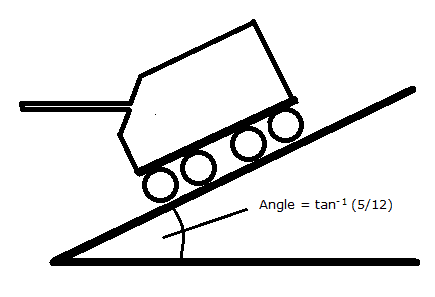# Help with variable mass on a slope question

• Nate-2016

## Homework Statement

An armoured car with a mass of 5 tonnes is located on a smooth plane which is inclined at an angle of tan-1 (5/12) to the horizontal as shown in Figure Q3. A missile of mass 15kg is fired horizontally from this armoured car at 650m/s. Determine the velocity with which the armoured car will begin to travel up the inclined plane.[/B]

## Homework Equations

I think that a momentum equation needs to be used, but I am not sure how to apply that equation to a slope.

## The Attempt at a Solution

The answer to the question is 1.8m/s. The closest I have got to it is 1.9m/s. This is my attempt.

0 = mdv - Mv
dv+v = 650m/s
mdv = Mv
mx(650-v) = Mv
15x(650-v) = 5000v
v = (15x650) / (15+5000)
v = 1.94 m/s

At which point do I taken into consideration the angle of the slope?

Whenever you choose.
When you draw up your equation, mdv = Mv, you are saying (I think?) that the momentum of the shell is equal to the momentum of the AC. Since v is a vector, you need to take the directions into account. But the shell is fired horizontally and the AC moves up the slope and you should take the angle into consideration here.
But you could consider momentum independently in horizontal and vertical directions. Then your equation is presumably looking only at the horizontal component of momentum and you have calculated the horizontal component of the AC velocity. So now you consider the slope.

I agree with your 1.95m/sec (I think you maybe got 1.96?) and I can see how someone might then get 1.8m/s by considering the slope, but I don't agree with that. I get 2.11m/sec for the AC velocity up the slope.

•Nate-2016
Thank you for explaining that to me. I have now taken into consideration the angle of the slope at the very end of my equation.
My new equation is now

0 = mdv - Mv
dv+v = 650m/s
mdv = Mv
mx(650-v) = Mv
15x(650-v) = 5000v
v = (15x650) / (15+5000)
v = 1.94 m/s x cos(tan^1(5/12))
v = 1.79 or 1.8 m/s

Solved.